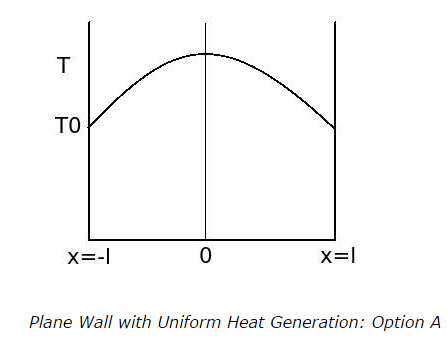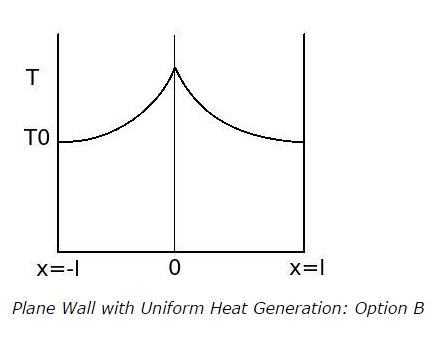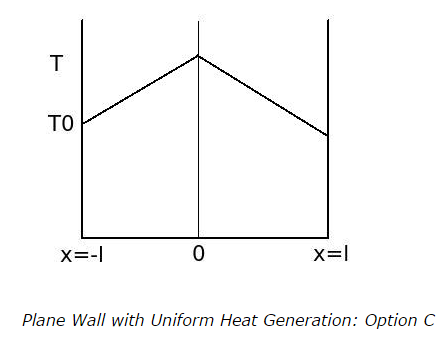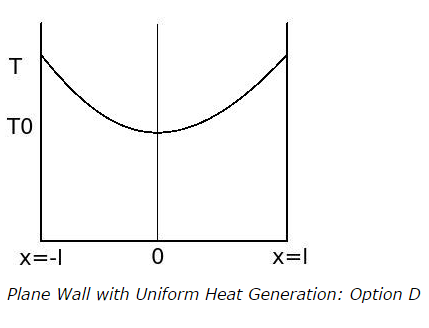# Steady-State Heat Conduction in Systems with Heat Generation - MCQs

## Steady-State Heat Conduction in Systems with Heat Generation – MCQs

1. Consider a plane wall of finite thickness having energy generation in it. The sum of the rate of heat energy generation in the wall and rate of energy transfer by conduction into the wall is equal to

a. rate of heat transfer by conduction out of the wall
b. rate of heat transfer by convection out of the wall
c. both a. and b.
d. none of the above

ANSWER: a. rate of heat transfer by conduction out of the wall

2. Which is the correct temperature profile in a homogeneous flat plate having thickness 2l with uniform heat generation for steady-state heat conduction?

Where,

x is a distance from the heat generation point

a.b.c.d.3. The maximum temperature difference between heat generation temperature (T) and the temperature of outer surface (To) of a plane wall of thickness 2l is given by

Consider,

Heat is generated at the rate of at the centre of wall being both the outer surfaces are at distance l from the heat generation point

k is thermal conductivity of the wall

a. (T – To)max = q̇l2 / k
b. (T – To)max = 2q̇l2 / k
c. (T – To)max = 4q̇l2 / k
d. (T – To)max = q̇l2 / 2k

ANSWER: d. (T – To)max = q̇l2 / 2k

4. What is the formula for maximum temperature (Tmax) at the center of a long and solid cylindrical electric wire of radius r?

Where,

is rate of heat generation into the electric wire
k is thermal conductivity of the electric wire
and To is the surface temperature of the wire

a. (Tmax)=(To) +(q̇r2/2k)
b. (Tmax) =(To) +(q̇r2/4k)
c.(Tmax) =(q̇r2/2k)
d. Tmax) =(To) +(4q̇r2/k)